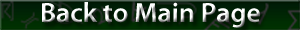Resistor Color Codes

#### Resistor Color Codes

Color coding is used to quickly determine the capacity or rating of an electronic component. For resistors, there are generally four color bands, with the following values:

• Band A is first significant figure of component value (left side)
• Band B is the second significant figure
• Band C is the decimal multiplier
• Band D, if present, indicates tolerance value in percent (no color means 20%)

The image here shows an example:Using the table below, this resistor would have a resistance of 2,700,000 ohms:

• The first band is red, which has a value of 2, and the second band is violet, with a value of 7, so the significant figure is 27
• The third band is green, which indicates a multiplier of 100,000, so the resistance value is 27 x 100,000, or 2,700,000 ohms
• The fourth band is gray, so this resistor's value is 2,700,000 ohms ±0.05%
Color Significant
figures
(Bands A and B)
Multiplier
(Band C)
Tolerance
Black 0 1 
Brown 1 10 ±1%
Red 2 100 ±2%
Orange 3 1,000 
Yellow 4 10,000 
Green 5 100,000 ±0.5%
Blue 6 1,000,000 ±0.25%
Violet 7 10,000,000 ±0.1%
Gray 8 100,000,000 ±0.05%
White 9 1,000,000,000 
Gold  .1 ±5%
Silver  .01 ±10%
None   ±20%